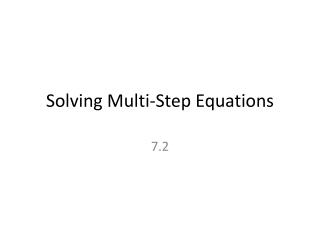DownloadDownload PresentationSolving Multi-Step Equations

# Solving Multi-Step Equations

Download Presentation## Solving Multi-Step Equations

- - - - - - - - - - - - - - - - - - - - - - - - - - - E N D - - - - - - - - - - - - - - - - - - - - - - - - - - -
##### Presentation Transcript

1. Useful Steps to Solve a Multi-Step Equations • Distribute (if necessary) • Combine like terms (if one same side of equation) • Undo addition or subtraction • Undo multiplication or division

2. 2x + 7 + x =16 3x+7 =16 3x =9 X=3

3. -3(m-6)=4 -3m +18 =4 -3m=-14 M =14/3

4. 3(x+12)-x=8 3x+36 –x =8 2x +36 =8 2x=-28 X=-14

5. 2(x+1) +x=7 2x+2 +x =7 3x +2 =7 3x =5 X= 5/3

6. Consecutive Numbers • Counting by ones from any integer • Examples: 120,121,122,… -5,-4,-3,… • Formula: n, n+1, n+2, n+3, n+4….

7. Consecutive Even Integers • Counting by evens from any integer • Examples: 2,4,6,8,… 122,124,126,128… • Formula: n, n+2, n+4, n+6, n+8, n+10

8. Consecutive Odd Integers • Counting by odds from any integer • Examples: …,-9,-7,-5,-3 83,85,87,89,… • Formula: n, n+2, n+4, n+6, n+8, n+10

9. Find four consecutive integers with a sum of 358 n + (n+1)+ (n+2)+ (n+3) = 358 4n+6 =358 4n =352 n =88 n+1=89 n+2=90 n+3=91 Answer: 88,89,90,91

10. Find two consecutive even integers with a sum of 66. n + (n+2) =66 2n+2 =66 2n=64 n=32 n+2=34 Answer:32, 34

11. Homework • Page 343 (11-29) all